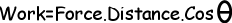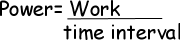Work;Power;Gravitational Potential Energy;

PE=mg.h m is the mass, g is the gravitational acceleration and h is the distance

Potential Energy of the Spring;

Ep=1/2.k.x² k is the spring constant and x is the amount of compression or streching

Kinetic Energy;

Ek=1/2mv² m is the mass, v is the velocity

Work Power Energy Exams and Solutions

Related